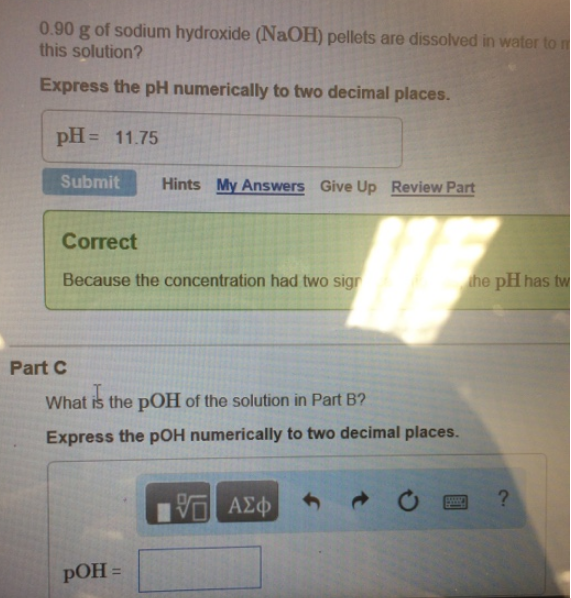# Problem: 0.90 g of sodium hydroxide (NaOH) pellets are dissolved in water to this solution? Express the pH numerically to two decimal places. pH = 11.75 What is the pOH of the solution in Part B? Express the pOH numerically to two decimal places.

###### FREE Expert Solution
91% (231 ratings)###### Problem Details

0.90 g of sodium hydroxide (NaOH) pellets are dissolved in water to this solution? Express the pH numerically to two decimal places. pH = 11.75 What is the pOH of the solution in Part B? Express the pOH numerically to two decimal places.